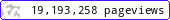•••••MadAsMaths.com :: I.Y.G.B. Practice Papers :: Undergraduate Methods Papers :: Mathematical Methods 4 Practice Papers

I.Y.G.B. MATHEMATICAL METHODS 4 PRACTICE PAPERS

These I.Y.G.B. practice papers follow the most common syllabi for a second year degree in Mathematics, Engineering or Science.

The examined topics are listed at the front cover of each paper.

All papers have model solutions.

 mathmeth4_a.pdfmathmeth4_a_solutions.pdfmathmeth4_b.pdfmathmeth4_b_solutions.pdfmathmeth4_c.pdfmathmeth4_c_solutions.pdfmathmeth4_d.pdfmathmeth4_d_solutions.pdfmathmeth4_e.pdfmathmeth4_e_solutions.pdfmathmeth4_f.pdfmathmeth4_f_solutions.pdfmathmeth4_g.pdfmathmeth4_g_solutions.pdfmathmeth4_h.pdfmathmeth4_h_solutions.pdfmathmeth4_i.pdfmathmeth4_i_solutions.pdfmathmeth4_j.pdfmathmeth4_j_solutions.pdfmathmeth4_k.pdfmathmeth4_k_solutions.pdfmathmeth4_l.pdfmathmeth4_l_solutions.pdfmathmeth4_m.pdfmathmeth4_m_solutions.pdfmathmeth4_n.pdfmathmeth4_n_solutions.pdfmathmeth4_o.pdfmathmeth4_o_solutions.pdfmathmeth4_p.pdfmathmeth4_p_solutions.pdfmathmeth4_q.pdfmathmeth4_q_solutions.pdfmathmeth4_r.pdfmathmeth4_r_solutions.pdfmathmeth4_s.pdfmathmeth4_s_solutions.pdfmathmeth4_t.pdfmathmeth4_t_solutions.pdfmathmeth4_u.pdfmathmeth4_u_solutions.pdfmathmeth4_v.pdfmathmeth4_v_solutions.pdfmathmeth4_w.pdfmathmeth4_w_solutions.pdfmathmeth4_x.pdfmathmeth4_x_solutions.pdfmathmeth4_y.pdfmathmeth4_y_solutions.pdfmathmeth4_z.pdfmathmeth4_z_solutions.pdf